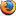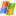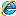### Perl 的四舍五入

```sub round{
my(\$value, \$rank) = @_;
if(\$value > 0){
return (int(\$value * 10**\$rank) + 0.5) / 10**\$rank;
}
else{
return (int(\$value * 10**\$rank) - 0.4) / 10**\$rank;
}
}
print round(12.345, 2);```

round() 函式的第一个参数为欲转换的数值，第二个参数为欲取得的小数点位数

### JavaScript 的四舍五入

```<script type='text/javascript'>
//方法一
function round1(value, rank){
if(value >= 0){
return parseInt(value * Math.pow(10, rank) + 0.5) / Math.pow(10, rank);
}
else{
return parseInt(value * Math.pow(10, rank) - 0.4) / Math.pow(10, rank);
}
}
//方法二: 使用内建的 round() 数学函数
function round2(value, rank){
return Math.round(value * Math.pow(10, rank)) / Math.pow(10, rank);
}
//方法三: 使用内建的 toFixed() 函数 (传回字串)
function round3(value, rank){
return Number.parseFloat(value).toFixed(rank);
}
console.log(round1(12.345, 2)); // 12.35
console.log(round2(12.345, 2)); // 12.35
console.log(round3(12.345, 2)); // "12.35"
</script>```

1.• 晴树
• 08/21. 2008 9:29上午
UsingMozilla Firefox 3.0 onWindows Vista

很不错的文章

像有些语言提供现成的round()，其实是‘四舍六入五成双’
判断方式就有点小差异

2.• cvictor
• 08/26. 2008 12:38上午
UsingMozilla Firefox 2.0.0.16 onWindows XP

负数的处理有点问题喔。还是要减0.5才对。

3.• 小迪
• 10/12. 2011 4:24下午
UsingInternet Explorer 8.0 onWindows XP

呵呵，0.5中间值